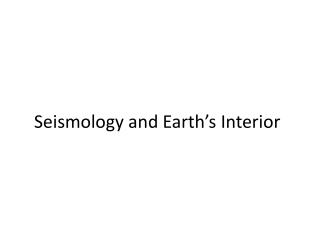DownloadDownload PresentationSeismology and Earth’s Interior

# Seismology and Earth’s Interior

Télécharger la présentation## Seismology and Earth’s Interior

- - - - - - - - - - - - - - - - - - - - - - - - - - - E N D - - - - - - - - - - - - - - - - - - - - - - - - - - -
##### Presentation Transcript

1. Seismology and Earth’s Interior

2. Mass of the Earth • Spherical masses behave as if all mass located at central point • g = GMe/R2 Me = gR2/G • g = 9.8 m/sec2 • R = 6,371,000m • G = 6.67 x 10-11 m3/(kg sec2) • Me = 9.8 x (6,371,000)2/(6.67 x 10-11) = 6 x 1024 kg

3. Mass in the Earth • Rotating bodies have a moment of inertia (I) • Corresponds to mass for linear motion • Rotational velocity used as velocity term • Generally of the form I = kMR2 • For a uniform sphere, k = 2/5 • For the earth, K = 0.33 • Hence, mass concentrated in center

4. Mass distribution in the Earth • Model Earth as series of thin shells with given density • Sum of masses must equal mass of the earth • Sum of moments of inertia must equal moment of inertia of the earth

5. Gravity inside a Sphere • Gravitational attraction inside a spherical shell = zero • Gravitational attraction inside a uniform sphere drops linearly to zero at the center • In the Earth: Gravity at radius r = Gmr/r2 • Mass above radius r has no effect • Surprise – nearly constant from surface to top of core • Pressure = weight of overlying shells • Thickness of shell x density x local gravity

6. How Seismographs Work

7. Seismic Waves

8. 1. Assume the Earth is uniform. • We know it isn't, but it's a useful place to start. It's a simple matter to predict when a seismic signal will travel any given distance.

9. 2. Actual seismic signals don't match the predictions • If we match the arrival times of nearby signals, distant signals arrive too soon • If we match the arrival times of distant signals, nearby signals arrive too late. • Signals are interrupted beyond about 109 degrees

10. 3. We conclude: • Distant signals travel through deeper parts of the Earth, therefore .. • Seismic waves travel faster through deeper parts of the Earth, and .. • They travel curving paths (refract) • Also, there is an obstacle in the center (the core).

11. Why Refraction Occurs

12. Waves Travel The Fastest Path

13. Seismic Waves in the Earth

14. Seismic Waves • P-Wave Velocity = /ρ = • S-Wave Velocity = =

15. Information Where We Need It Most

16. Properties of the Deep Earth • P- and S-wave Velocities give us E, everywhere in the Earth • Know density and pressure from mass-balance and moment of inertia • Know composition from meteorites and mantle xenoliths • Compare mechanical properties and density to rocks

17. Continental Drift is Impossible! • Shear modulus of deep mantle is 300 Gpa: more than steel • Therefore continental drift is impossible! • Fallacy: failure to recognize time scales • Seismic waves reflect properties on a scale of seconds • Convection reflects properties on a scale of years

18. Travel Time Curve

19. Inner Structure of the Earth

20. The overall structure of the Earth

21. Locating Earthquakes

22. Locating Earthquakes

23. Locating Earthquakes

24. Locating Earthquakes - Depth

25. Temperature: The Hard Part • Near surface gradient = 25K/km • Center of Earth would be 160,000 K at that rate • We know mantle is solid (transmits S-waves) • Estimated mostly from experimental data • Extrapolating melting points to high pressure • Comparing observed elastic properties with lab data